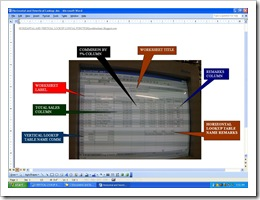# Vertical Lookup and Horizontal Lookup Application of MS-ExcelVERTICAL LOOKUP AND HORIZONTAL LOOK UP TABLE is one of the logical calculation of MS-Excel application of MS-office throughout the different versions.This is useful for creating a worksheets for monthly commissions of the salesman.

Now, in creating this worksheets you need to know what are the labels you need for your worksheets.So, therefore you can calculate how much the number of columns and rows you need in this application.Then create the border of the entire worksheets and align the worksheets label.Then input carefully the data by columns of the different labels.One of the most very sensitive is inputting or typing the different figures.Because this application base mostly of the figures found in this worksheets.To avoid this error,once you type the figures,do not put comma and dot using your keyboard.Use the formatting bar located at the top of your worksheets by highlighting your entire figures and click the comma or dot button.

Now, as what you see in the picture of this post,I have vertical lookup table and horizontal table for the need to comply this application.So before to begin the steps how to create this worksheets.Please just analyze first this logical formula.

1. For the vertical lookup formula, this is only an example:
=vlookup(c8,comm,2)
The component of logical formula
1. =vlookup- this is the logical function name vertical lookup
2. c8- the reference data(c is the column header and 8 is the row header of the worksheets)
3. comm- name of the vertical table
4. 2- is the column index number(number of columns of the vertical table)
2. For the horizontal lookup formula, this is only an example:
=hlookup(j8,remarks,2)
The component of logical formula
1. =hlookup- this is the logical function name horizontal lookup
2. j8- the reference data
3. remarks- name of the horizontal table
4. 2- row index number
Note: Use the open and close parenthesis to enclose the relative data and use comma to separate each.
Now here are the steps:
1. Create the worksheets table according to the requirements you need in your worksheets.
2. Input carefully the data according to its worksheets label(Be assure of the number of figures).
3. Create your vertical table(See the picture of this post).
4. Highlight the entire vertical table.
5. Click the namebox, then type the name of the table.Do not forget to press enter key to apply the name.
6. Now, you are ready to apply the logical formula of the vertical lookup by positioning the worksheet cell below of your worksheet label name commission by 5%.
7. Then press enter key once you finish typing the formula.
8. Then you will see the results, the results will defend what are the conditions  you give, ex: if sales is 0-45001 commission is 5%, if sales is 45001- 65001 commission is 10%, and if sales is 70000 above commission is 15%.
9. Then, use autofill tab of the worksheet cell of the proceeding data.By dragging your mouse using the black crosshair of the cursor of the worksheets.
10. Now, if you want to calculate the total commission.Just use this formula, ex: =c8*d8, then press enter key.Then use autofill tab of your worksheet cell to calculate the proceeding data.Note: the formula is just only an example,look at your reference data to calculate exactly.(see the picture above)
11. Then, now you are ready to calculate the gross pay by adding the total commission and net pay, here is the formula,ex: =e5+f5, then press enter key.
12. Now, create the horizontal lookup table by percentage.And below of it,type the logical conditions(see the picture above)
13. Then, click the name box again then type the name of the table, ex: Remarks, then press enter key.
14. Now, you are ready to input the formula of the remarks column of your worksheets.Position your worksheet cell below of the remarks label
15. Then, type the formula of the horizontal lookup(see the above of this post),then press enter key.
16. Now, you will see the logical quoted text base in your logical data conditions by percentage.
17. Then, use autofill tab, to calculate the proceeding data.

“That’s all again, just type in the comment bar if you have any question regarding our application”